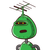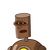# in ∆PQR, angle PQR =90° .QS perpendicular to PR and ST perpendicular to QR. if PQ =6 cm and PS =3 cm, find the area∆QST/area∆RST​

in ∆PQR, angle PQR =90° .QS perpendicular to PR and ST perpendicular to QR. if PQ =6 cm and PS =3 cm, find the area∆QST/area∆RST​

### 2 thoughts on “in ∆PQR, angle PQR =90° .QS perpendicular to PR and ST perpendicular to QR. if PQ =6 cm and PS =3 cm, find the area∆QST/area∆RST​”

1.Steps and Understanding :

1) In PQS,

cos (P) = PS/PQ

=> cos(P) = 3/6 = 1/2

=> P = 60°

2) Now, finding other angles as shown in pic by using properties of triangle.

3) In PQS,

tan(60°) = QS/PS

=> QS = 3* tan(60°) = 3√3cm

In QST,

sin(60°) = ST/QS

=> ST =( 3√3) * (√3/2 )

=> ST = 9/2 cm.

cos(60°) = QT/QS

=> 1/2 = QT/QS

=> QT = 3√3 * (1/2)

=> QT = 3√3/2 cm

4) In RST,

tan(30°) = ST/TR

=> 1/√3 = (9/2)/ TR

=> TR = 9√3/2 cm.

5) Now, Finally

area ( QST) / area (RST) =

( 1/2 * QT* ST )/( 1/2 * RT* ST)

=QT/RT

(3√3/2)/(3√3/2)

=1/3

2.